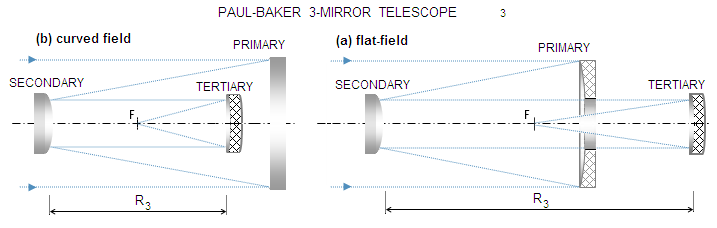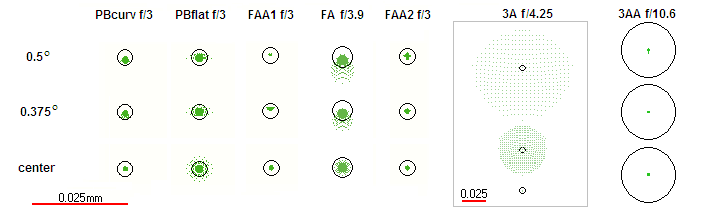telescopeѲptics.net          ▪▪▪▪                                             CONTENTS

# 8.3.1. Paul-Baker and other three-mirror anastigmatic aplanats

Paul-Baker three-mirror system combines concept of the Mersenne telescope with the unique property of a sphere to be free from primary coma and astigmatism with the aperture stop at the radius of curvature. The basic, curved field PB concept, uses a pair of confocal mirrors - concave paraboloidal primary and convex spherical secondary - with the third, concave spherical mirror placed so that the secondary vertex coincides with its center of curvature. In effect, the secondary acts as an aperture stop for the tertiary (FIG. 129). As long as radii of curvature of the tertiary and secondary are identical, their spherical aberration contributions are also identical and of opposite sign, and the system is free from primary spherical aberration, as well as from coma and astigmatism.FIGURE 129: Paul-Baker three-mirror anastigmatic aplanat. The basic arrangement (left) consists from a concave paraboloidal primary (P), convex spherical secondary (S) and concave spherical tertiary (T). The only remaining third-order aberration is field curvature, which can be corrected by extending secondary-to-tertiary separation and aspherizing the secondary (right). Secondary in either arrangement is at the center of curvature of the tertiary mirror.

The parameters are exceptionally simple for a three-mirror telescope: the system is fully specified by the above description for the primary of ~/3 and slower. Faster PB systems require higher-order aspherics added to one or more surfaces for optimum performance. The PB image curvature is Rp=R1/2, R1 being the radius of curvature of the primary, with the curvatures of the secondary and tertiary cancelling each other.

The PB flat-field variant also uses paraboloidal primary and spherical tertiary. Primary-to-secondary separation is given by S1=(1-k)R1/2, with the secondary conic K2=-1+(1-k)3 and the radius of curvature R2=kR1. Distance to the tertiary - and tertiary radius of curvature - is S2=R3=kR1/(1-k). The k parameter is the height of the marginal ray at the secondary (and tertiary), in units of the aperture radius. It is also the secondary-to-primary-focus separation in units of the primary's focal length. In either case, it is determined by the secondary location, which is arbitrary (except for the small/large extremes). The tertiary is behind primary for k>0.268; for k=0.268 the two mirror centers coincide, and for smaller k values tertiary is located between primary and secondary.

The FAA1 and FAA2 are also relatively simple systems, rivaling the Paul-Baker in image quality. The FAA1 consists of the near-paraboloidal (ellipsoidal) primary and a pair of spherical mirrors, as shown on FIG. 130a. A pair of spherical mirrors in this arrangement can also work with paraboloidal primary, but can't correct all four 3rdFIGURE 130: (a) FAA1, flat-field anastigmatic aplanat consisting from: (1) concave ellipsoidal primary, (2) small convex spherical secondary outside the primary focus, and (3) concave spherical tertiary. The field is somewhat more limited in size than in either Paul-Baker or FAA2, due to vignetting caused by restricted tertiary size. Configuration can't vary significantly, but the system /ratio is very flexible. Excellent performance extends to ~/3, without a need to add higher-order surface aspherics (in the range of amateur apertures).

(b) FAA2, a flat-field anastigmatic aplanat consisting from: (1) concave ellipsoidal primary, (2) small convex spherical secondary placed inside the primary focus, and (3) concave ellipsoidal tertiary. Effective central obstruction achievable with this design is significantly smaller than with either Paul-Baker of FAA1. This makes it viable for visual observing, after a diagonal flat is added to make the final image accessible. Not quite as well corrected as the FAA1 at very large relative apertures, but the difference is of no practical significance. First published by Korsch in a very similar form.

order aberrations (leaving distortion out). Very low residual coma remains present. Required system parameters are more complex than those for the PB. For that reason, only actual prescriptions are given for the main FAA1 arrangement, the anastigmatic aplanat, and the version with paraboloidal primary, with slight residual coma (the parameters scale with the aperture, or with the primary radius of curvature).

- FAA1, flat-field anastigmatic aplanat:
σ1=0.54515,  σ2=-0.11423,  ρ2=0.14433,  ρ3=0.16907,  K1=-0.95,  K2,3=0

- FA, flat-field anastigmat:
σ1
=0.5429,  σ2=-0.1115,  ρ2=0.1429,  ρ3=0.1666,  K1=-1,  K2,3=0

- AP, aplanat:
σ1=0.544,  σ2=-0.113,  ρ2=0.12,  ρ3=0.167,   K1=-1,  K2,3=0

where σ1 and σ2 are primary-to-secondary and secondary-to-tertiary separation, respectively, and ρ2 and ρ3 are secondary and tertiary radius of curvature, respectively, all expressed in units of the primary radius of curvature. The effective system focal length is typically somewhat (up to ~10%) smaller than primary's f.l. The parameters should give near-optimum performance; it can be maintained within a small degree of compensatory changes in parameters (for instance, the aplanat gives nearly identical performance with the secondary-to-tertiary distance changed to -0.11R, and tertiary radius to 0.164R)

The FAA2 uses concave ellipsoidal primary, convex spherical secondary forming the focus between the two mirrors, and concave ellipsoidal tertiary placed behind the primary (FIG. 130b). It is very similar to Korsch's three-mirror flat-field anastigmatic aplanat, in which the secondary is hyperboloidal (there is no need for aspherizing in my version). Mirror positioning is similar to the flat-field PB, but mirrors are somewhat easier to make, baffling is better and central obstruction required for a given field is smaller. Correction of primary aberrations is even slightly better than in the PB, but the difference has no practical consequences. An actual system prescription, with the above notation, is as follows:

σ1=0.41896,  σ2=-0.70833,  ρ2=0.25,  ρ3=1/3,  K1=-0.7196,  K2=0  and  K3=-0.3496

The effective system focal length is typically ~50% longer than that of the primary. In setting up the design, a near flat-field condition requires near-zero Petzval, which is achieved by selecting needed radii of curvature of the secondary and tertiary for given primary. Spherical aberration is controlled with the primary conic, coma by the primary-to-secondary separation, and astigmatism by the secondary-to-tertiary separation (the former influences coma much more than astigmatism, the latter the other way around, so it takes a few steps to have them optimally balanced).

FIG. 131 illustrates performance of the above systems with the ray spot plot over 1 degree field diameter.FIGURE 131
: 1o field ray spot plots for (left to right) curved and flat-field Paul-Baker (PB), anastigmatic aplanats FAA1 and FAA2 (FA is FAA1 versions with parabolic primary), and folded Cassegrain-Gregorians, 3A (reduced by a factor of 4 to fit in) and 3AA. Aperture diameter is 300mm for all except 3AA (D=400mm). At �/3, higher order spherical becomes noticeable, in particular in the flat-field PB variant (requires higher-order aspheric term surface correction). Note that the angular field for 3AA is different than for the rest of systems, 0, 0.23 and 0.33 (for 25mm field radius); also, spots for the PB (curved field), 3A and 3AA are on best curved image surface, with the radii -900mm, 333mm and -240mm, respectively.               SPEC'S

As mentioned, visual field quality in the Cassegrain-Gregorian can be expected to be significantly better, due to its astigmatism partly cancelling that of the eyepiece. For instance, the RMS error of the above CG at 0.5o off-axis is some 2.5 times the error of a comparable Newtonian in the image produced by the objective. With a typical 30mm Kellner - which still has a few times stronger astigmatism, opposite in sign to that in the CG - the resulting CG RMS error is about 25% smaller than that in the Newtonian. With modern eyepiece types better corrected for astigmatism, nearly full field correction at a certain eyepiece f.l. is possible.

In principle, similar field correction effects should be expected in combination with a focal reducer lens.